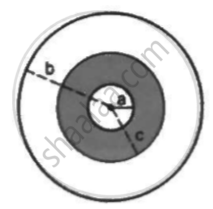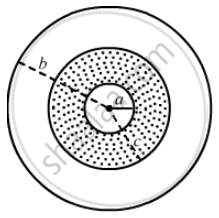Department of Pre-University Education, KarnatakaPUC Karnataka Science Class 12
Share

# A Sphercial Capacitor is Made of Two Conducting Spherical Shells of Radii a and B. the Space Between the Shells is Filled with a Dielectric of Dielectric Constant - Physics

ConceptCapacitors and Capacitance

#### Question

A sphercial capacitor is made of two conducting spherical shells of radii a and b. The space between the shells is filled with a dielectric of dielectric constant K up to a radius c as shown in figure . Calculate the capacitance.#### SolutionWe have two capacitors: one made by the shells a and c and the other made by the shells b and c.

The capacitance of the capacitor C_(ac) is given by

C_(ac) = (4pi∈_0acK)/((c-a))

The capacitance of the capacitor C_(cb) is given by

C_(cb) = (4pi∈_0bcK)/(K(b-c)

The two capacitors are in series; thus, the equivalent capacitance is given by

1/C = 1/C_(ac) + 1/C_(cb)

⇒ 1/C = ((c-a))/(4pi∈_0acK) + ((b-c))/(4pi∈_0cb)

⇒ 1/C = (b(c-a)+Ka(b-c))/(K4pi∈_0abc)

⇒ C = (K4pi∈_0abc)/(b(c-a)+Ka(b-c))

Is there an error in this question or solution?

#### Video TutorialsVIEW ALL 

Solution A Sphercial Capacitor is Made of Two Conducting Spherical Shells of Radii a and B. the Space Between the Shells is Filled with a Dielectric of Dielectric Constant Concept: Capacitors and Capacitance.
S